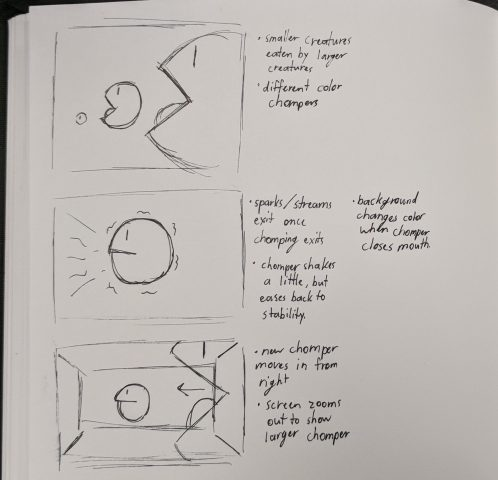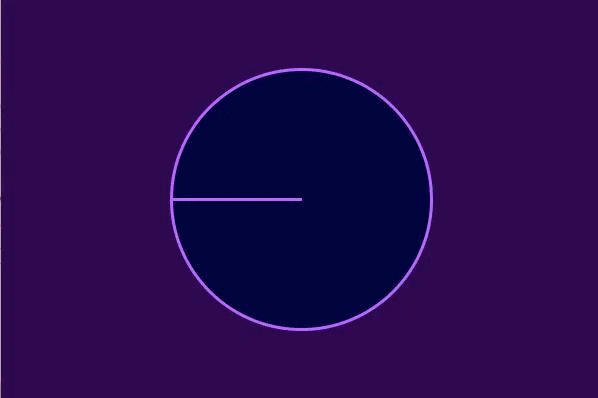# farcar – AnimatedLoop

One More Bite (2018)

SketchAnimated GIFAnd a square version too…Description

One More Bite was written in Processing with the help of Golan Levin’s Pattern_Master Library. PennerEaseOutExpo as well as custom easing based on cosine waves were used for fluid motion. These functions allowed for sharp, gradual accelerations that I was looking to use during the sketching phase. As a simple, looping  GIF, this product met my expectations for the most part. The fluidity and color scheme are what I envisioned. A limitation of mine was when trying to stylize the background to a grid-like environment, the program would lag. I had to remove this feature despite my efforts to make it run fasted because, in the case of this animation, the quality is more in the motion than the design. This goes to say that an improvement i could have made would be to design more complex characters and backgrounds without having the program lag as it did when I played with the background.

Code

```float time, scale, xs, ys, pos, mouth; int inst, num; PVector posC; Colour data[] = new Colour;;   //Colour class to hold background, fill, and stroke colors for a given inst public class Colour { public color bg; public color fl; public color str;   public Colour(color bg_1, color fl_1, color str_1) { bg = bg_1; fl = fl_1; str = str_1; } }     void setup(){ size(600,400); time = 0; scale = 1; xs = 0; ys = 0; pos = 0; mouth = 0; posC = new PVector(0,0); data = new Colour(color(46,8,81),color(2,5,61),color(184,104,255)); data = new Colour(color(9,89,83),color(6,61,57),color(84,249,237)); data = new Colour(color(96,12,70),color(73,8,53),color(242,79,192)); data = new Colour(color(119,82,19),color(94,64,14),color(237,177,75)); num = 4; smooth(); }   void draw(){ if(time&gt;52) background(data[(inst+1)%num].bg); else background(data[inst%num].bg); //grid(); fill(data[inst%num].fl); stroke(data[inst%num].str); loop(); time++; if (time&gt;70) { setup(); inst++; } }   //draws a bg grid texture //WARNING: makes program run slower void grid() { noStroke(); fill(255); for(int i = 0; i &lt; 605; i+=5) for(int j = 2; j &lt; 405; j+=5) ellipse(i,j,1,1); }     //animation loop void loop() { pushMatrix(); translate(width/2, height/2); //zooms out if (time>20 && time < 30) scale = 1 - 0.68454*function_PennerEaseOutExpo(map(time,20,30,0,1));   //squashes chomper if (time>40 && time<47) { float temp = time-40; xs += 2*temp*temp; ys -= 2*temp*temp; }   //restores chomper if (time>47 && time<54) { float temp = time-47; xs -= 2*temp*temp; ys += 2*temp*temp; }   //opens chomper mouth if (time>40 && time<54) mouth += 0.32*cos(map(time,40,54,0,PI));   //moves chomper if (time>40 && time<50) pos = 8*(time-40)*(time-40)+20;   //shakes chomper if (time>50 && time<60) { float temp = 45/function_PennerEaseOutExpo(map(time,50,60,0,1)); posC.x = random(-temp,temp); posC.y = random(-temp,temp); }   //generates lines if(time>50 && time<70) { stroke(data[(inst+1)%num].str); float temp = 4; if(time>63) temp = 35-0.5*time; strokeWeight(temp); float start = 50+180*function_PennerEaseOutExpo(map(time,50,70,0,1)); float end = 200+30*function_PennerEaseOutExpo(map(time,50,70,0,1)); lineByAngle(0,0,PI-0.5*QUARTER_PI,start,end); lineByAngle(0,0,PI,start,end); lineByAngle(0,0,PI+0.5*QUARTER_PI,start,end); float start_small = 100+90*function_PennerEaseOutExpo(map(time,50,70,0,1)); float end_small = 160+30*function_PennerEaseOutExpo(map(time,50,70,0,1)); strokeWeight(2); lineByAngle(0,0,PI-0.25*QUARTER_PI,start_small,end_small); lineByAngle(0,0,PI+0.25*QUARTER_PI,start_small,end_small); stroke(data[inst%num].str); } //resets chomper position if (time>60 && time<70) { posC.x -= 0.5*posC.x; posC.y -= 0.5*posC.y; } //draws shapes scale(scale); strokeWeight(12-9*scale); arc(0, 0, 260, 260, PI, PI+2*PI, PIE); fill(data[(inst+1)%num].fl); stroke(data[(inst+1)%num].str); arc(1.05*width+120*scale-pos+posC.x, posC.y, 819.85+xs, 819.85+ys, PI+mouth, PI+2*PI-mouth, PIE); fill(data[inst%num].fl); stroke(data[inst%num].str); popMatrix(); }     void lineByAngle (float x, float y, float r, float start, float end) { line(x+start*cos(r),y+start*sin(r),x+end*cos(r),y+end*sin(r)); }     //Easing function courtesy of Golan Levin. //----------------------------------------   float function_PennerEaseOutExpo(float t) { return (t==1) ? 1 : (-pow(2, -10 * t) + 1); }```